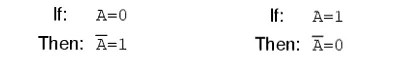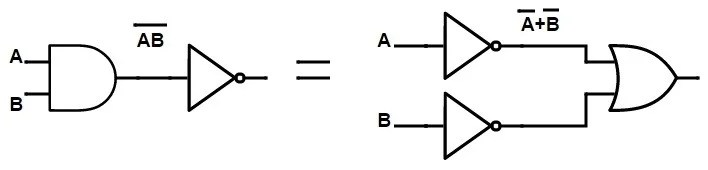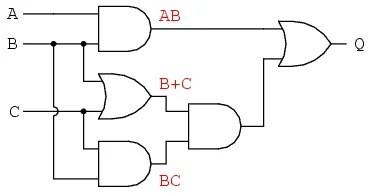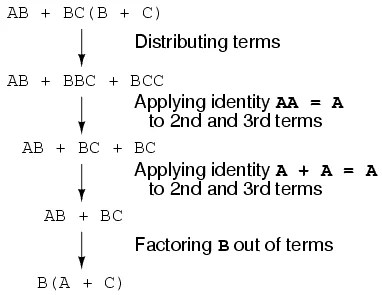View Course Path

# Boolean Algebra – All the Laws, Rules, Properties and Operations

Boolean algebra is a branch of algebra wherein the variables are denoted by Boolean values. True (also represented by a 1) and False (also represented by a 0). That’s it. Those are the only two values we’ll deal with in Boolean algebra or digital electronics for that matter. Boolean algebra differs from the mathematical algebraic system with respect to the operations done on its variables. Since this is a new system, there are some new rules and laws that apply. Let’s check those out.

Here's some help to help you visualize what Boolean algebra means.

You wake one morning with the sunshine falling on your face. You drowsily walk to your coffee maker. What is this you see? 0s and 1s on the machine? What happened to your coffee maker? You open your mouth to exclaim your surprise. But all you can utter is ‘Yes’. And ‘No’. That’s it. Your vocal cords support no other words.

You rub your eyes and look around your room. Everything in the room – from your TV remote to your motivational posters, everything has just two words on them. What the heck!

After the initial panic attack spurred by the changed atmosphere, you realize that the world is now a simpler place. A plain combination of just two values powers every system. It’s just you, your ‘Yes’ and ‘No’.

That’s pretty much the world of digital electronics. And binary is the language of this world. This language is governed by Boolean algebra. And that’s what we will understand in this post. Once we know Boolean, we can just look at an electronic circuit’s equation and visualize its design and behavior.

Back to the world of more than two digits.

The main operations performed on Boolean algebra are conjunction (Boolean AND), disjunction (Boolean OR) and negation (Boolean NOT). The OR function is similar to binary addition, whereas the AND function is similar to binary multiplication.

The AND operation is denoted by Λ, OR operation is denoted by , and a ¬ denotes the NOT operation. Alternatively, a (×), (+) and a ( ¯ ) denotes the AND, OR and NOT operations, respectively.

In digital electronics, circuits involving Boolean operations are represented in Boolean expressions. Boolean algebra helps in simplification of a given logic expression without altering any functionality of any operations or variables.

Contents

## History of Boolean Algebra

Aristotle’s system of logic was given a new face, using symbolic forms introduced by English mathematician George Boole. Boole introduced several relationships between the mathematical quantities that possessed only two values: either True or False, which could also be denoted by a 1 or 0 respectively. This system was later devised as Boolean Algebra. The results of all mathematical operations performed on these values could also possess only two values: 1 or 0.

## Why do we need Boolean Algebra to reduce logical expressions?

Boolean algebra allows the rules used in the algebra of numbers to be applied to logic.  It simplifies Boolean expressions which are used to represent combinational logic circuits.

It also helps in minimizing large expressions to equivalent smaller expressions with lesser terms, thus reducing the complexity of the combinational logic circuit it represents, using lesser logic gates for the circuitry. Additionally, reducing the size of the circuitry also increases the speed of the circuit.

Furthermore, a decrease in the number of logic gates reduces the power dissipation in the circuit. This provides us with a minimized, optimum circuit for a given logic.

In summary
Boolean algebra is used to simplify the complex logic expressions of a digital circuit. Thereby allowing us to reduce complex circuits into simpler ones. It goes something like this. Complex circuit -> Find equation -> Reduce using Boolean laws -> Redesign circuit based on new simpler equation.

Next up, let’s check out the basic functions of Boolean algebra.

## NOT Operation and its rules

A Boolean NOT (!) performs an inversion function. The NOT operation is called so because the output is NOT the same as the input.

Applying the NOT operation to a ‘True’ variable results in a ‘False’ output. Similarly, applying the NOT operation to a ‘False’ variable results in a True output. It can be compared with a simple NOT gate, which inverses/complement the input of a logic ‘1’ to a logic ‘0’, and vice versa.### Complement Law

When an OR operation is performed between a variable and its complement, the result is 1. Similarly, when an AND operation is performed between a variable and its complement, the result is 0.

 A + A’ = 1 A A’ RESULT 0 1 1 1 0 1
 A . A’= 0 A A’ RESULT 0 1 0 1 0 0

### Double Negation Law

The law basically says that if you use the NOT operation twice on a variable, you get back the original variable without any change in its value. Consider a variable A. Let the negation of A, i.e. A’ be given by Y. If we perform the negation operation on Y, we get back the variable A.

 (A’)’= A A A’=Y (A’)’ 0 1 0 1 0 1

## AND Operation and its rules

An AND operation results True if all its variables in the Boolean expression are True. If either of the variables in the expression is False, the result is False.

 A . B = Y A B Y 0 0 0 0 1 0 1 0 0 1 1 1

The AND operation follows a few rules/properties/laws on its functionality, namely the Annulment law, Identity property, Idempotent property, Complement property, and Commutative property. Let us consider A to be a Boolean variable, possessing the value of either a 0 or 1.

A . 0 = 0

A . 1 = A

A . A = A

A . A’ = 0

## OR Operation and its rules

An OR operation results True if either of its variables in the Boolean expression is True. If all the variables in the expression are False, the result is False.

 A + B = Y A B Y 0 0 0 0 1 1 1 0 1 1 1 1

Like the AND operation, the OR operation also follows a few laws on its functionality. Namely the Annulment law, Identity property, Idempotent property, Complement property, and Commutative property. Let us consider A to be a Boolean variable, possessing the value of either a 0 or 1.

A + 1 = 1

A + 0 = A

A + A = A

A + A’ = 1

## Distributive Laws of Boolean Algebra

There are two statements under the Distributive Laws:

### Statement 1

Consider three variables A, B, and C. When two variables are ANDed and ORed with a third variable, the result is the same as ORing the first and second variable with the third variable separately, and then ANDing their result.

In simple words, the product of two variables, when added to a third variable, produces the same result as when we add each variable with the third variable separately and multiply their sums.

A + ( B . C ) = ( A + B ) . ( A + C )

Here, the OR distributes over the AND operation.

PROOF

A + (B.C) = (A . 1) + (B.C)                              [A.1 = A by the Identity Property of AND]

= (A .(1 + B))+ (B.C)                                       [1 + B = 1 by the Annulment Property of OR]

=  (A.1) + (A.B) + (B.C)

= (A . (1 + C)) + (A.B) + (B.C)

= A .(A + C) + B.(A + C)                                  [A.A = A.1 = A]

= (A + C).(A + B)

Hence, the distributive law holds true.

### Statement 2

Consider three variables A, B, and C. When two variables are ORed and ANDed with a third variable, the result is the same as ANDing the first and second variable with the third variable separately, and then ORing their result.

In simple words, the sum of two variables, when multiplied to a third variable, produces the same result as when we multiply each variable with the third variable separately and add their products.

A.(B+C) = (A.B) + (A.C)

Here, the AND distributes over the OR operation.

PROOF

A . (B + C) = A.(B.1) + A.(C.1)               [1.B = B, 1.C = C by Identity Property of AND]

= [(A.B) . (A.1)] + [(A.C) . (A.1)]

=[ (A.B) . A ] + [ (A.C) .A ]

= ( A +1 ). [(A.B) + (A.C)]

= (A.B +A.C)                                            [1 + A = 1 by the Annulment Property of OR]

Hence, the distributive law holds true.

## Commutative Laws of Boolean Algebra

The Commutative law states that inter-changing the order of operands in a Boolean expression has no effect on its result.

A + B = B + A

A . B = B . A

## Associative Laws of Boolean Algebra

There are two statements under the Associative Laws:

### Associative Law using OR function

Associative law using the OR function states that ORing more than two Boolean variables will return the same output, irrespective of the order of the variables in the equation and their grouping. No matter which order the variables are swapped in, ORing them will always give the same result.

P + ( Q + R ) = ( P + Q ) + R

### Associative Law using AND function

Associative law using the AND function states that ANDing more than two Boolean variables will return the same output, irrespective of the order of the variables in the equation and their grouping. No matter which order the variables are swapped in, ANDing them will always give the same result.

P . ( Q . R ) = ( P . Q ) . R

## Absorption Property

This property ‘absorbs’ variables in a Boolean expression, thus reducing the complexity of the expressions to a simples one.

### OR Absorption Law

A + ( A . B ) = A

PROOF

A + ( A . B ) = (A . 1) + ( A . B)            [A.1 = A by the Identity Property of AND]

=A (1+B)

= A.1                                                     [1 + B = 1 by the Annulment Property of OR]

= A

### AND Absorption Law

A . (A + B) = A

PROOF

A . (A + B) = (A . A) + (A . B)                [Distributive Property]

= A + (A . B)                                          [A.A = A by Idempotent Property of AND]

= A (1 + B)

= A.1                                                      [1 + B = 1 by the Annulment Property of OR]

= A

## Precedence of Logical Operations in Boolean Algebra

When you solve Boolean expressions, multiples operators are used in the expressions. Which operator to be used first, which operator should be used next might be a confusing issue.

The highest precedence operator in an expression is grouped with the variables first and evaluated first, and then the next highest precedence operator is grouped with the remaining variables, and thus it goes on. Assuming there are many operators of the same precedence in an equation, the Boolean expression is then evaluated from left to right.

Irrespective of the operators in the equation, the parentheses are always given the utmost priority while solving equations.

 OPERATOR SYMBOL PRECEDENCE NOT ! Highest AND . Medium OR + Lowest

## De Morgan’s Theorem

Augustus De Morgan devised the De Morgan’s laws for Boolean expressions. These are two laws that help in simplifying or solving the Boolean equations.

### Statement 1

‘The negation of a disjunction is the conjunction of the negations,’ i.e. NOT (A OR B) = NOT A AND NOT B. It can also be stated as:

‘The complement of the union of two sets is the same as the intersection of their complements.’

###Statement 2

‘The negation of a conjunction is the disjunction of the negations,’ i.e. NOT (A AND B) = NOT A OR NOT B. It can also be stated as:

‘The complement of the intersection of two sets is the same as the union of their complements.’

##Duality Principle

The dual of a Boolean expression can be obtained by replacing all the AND operators to OR and all the OR operators to AND, and by replacing all the binary values, i.e. all the 0 with 1 and all the 1 with 0 in the equation.

The basic steps to be followed while following the Duality principle are:

• Change all the AND operators to OR operators
• Change all the OR operators to AND operators
• Complement all the 1s to 0s
• Complement all the 0s to 1s
 VALUE DUAL OR operator AND operator AND operator OR operator 1 0 0 1 A A’ A’ A

## Redundancy / Consensus Theorem

The Redundancy Theorem, also known as the Consensus Theorem, can be used as a trick in simplifying/reducing Boolean expressions and solving it. There are four simple criteria which can be used in reducing the equations:

• The expression must have three variables
• Each variable must be repeated twice, even though it is in its complemented form
• Only one out of the three variables must be in its complemented form
• For reduction, consider the terms containing the variable which has been complemented

The term which is omitted is called the consensus of the other two terms.

Here we have an example of the Redundancy Theorem with its proof.

Let Y = AB + A’C + BC be the given equation. Let us see if it agrees to the given criteria of the Consensus theorem.

• The given equation Y has three variables A, B, and C.
• Each variable A, B, and C is repeated twice, even though A is complemented.
• Only one variable, i.e. A is complemented in the equation
• Consider the terms where A is present, as A is the complemented term.

Thus, the reduced equation is Y = AB + A’C. BC is the consensus of the terms AB and A’C.

Let us now check the proof.

Y = (A . B) + (A’ . C) + (B . C)

= (A . B) + (A’ . C) + (B . C . 1)

= (A . B) + (A’ . C) + (B . C . (A + A’))                   [A + A’ = 1 by the Complement Property of OR]

= (A . B) + (A’ . C) + (A .B . C ) + (A’ . B . C)

= (A . B) (1 + C) + (A’ . C) (1 + B)                          [1 + B = 1 + C =1 by the Annulment Property of OR]

Y = (A . B) + (A’ . C)

Thus, redundancy theorem helps in simplifying Boolean expressions. Let us check a few more examples and apply the four criteria and figure out the answer.

•  F = (A + B) . (A’ + C) . (B + C)
1. The given equation F has three variables A,B and C.
2. Each variable A, B and C is repeated twice, even though A is complemented.
3. Only one variable, i.e. A is complemented in the equation
4. Consider the terms where A is present, as A is the complemented term.

Thus, F = (A + B) . (A’ + C)

• G = (P . Q’) + (P . R) + (Q . R)
1. The given equation G has three variables P,Q and R.
2. Each variable P, Q and R is repeated twice, even though Q is complemented.
3. Only one variable, i.e. Q is complemented in the equation
4. Consider the terms where A is present, as A is the complemented term.

Thus, G = (P . Q’) + (Q . R)

• Y = (D’ + F) . (E’ + F’) . (D’ + E’)
1. The given equation Y has three variables D’ ,E’ and F’.
2. Each variable D’, E’ and F’ is repeated twice, even though F’ is complemented.
3. Only one variable, i.e. F’ is complemented in the equation
4. Consider the terms where F’ is present, as F’ is the complemented term.

Thus, Y = (D’ + F) . (E’ + F’)

• Z = (A . B) + (B . C’) + (A . C)
1. The given equation Z has three variables A,B and C.
2. Each variable A, B and C is repeated twice, even though C is complemented.
3. Only one variable, i.e. C is complemented in the equation
4. Consider the terms where C is present, as C is the complemented term.

Thus, Z = (B . C’) + (A . C)

Interesting? Try out one problem yourself and give your answers in the comments section! Explain the reason as well for your answer!

N = (P + R) . (Q + R’) . (P + Q)

## Converting Logic Circuits to Boolean Expression Equivalents – Example

Imagine we have a large system of circuits with many logic gates. The aim is to convert this large circuit into its equivalent Boolean Expression. But where do we begin from? Which gate do we start from? How to write down the final output?

Well. We can easily write Boolean Expressions by converting the large circuit into smaller subsystems, considering each gate to be a subsystem. Write down the output of each gate corresponding to the signals given as input to the gate. OR gates are equivalent to Boolean addition, and AND gates are equivalent to Boolean multiplication. Always start from the left and go step by step towards the rightmost gate, considering the previous outputs from the left-side gates.

Step 1Step 2Step 3Now that you have the final expression check if there is a possibility of simplifying the equation. If not, this is the Boolean expression equivalent of the given logic circuit!The output of the circuit was (A . B) + (B . C).(B + C), but we could further simplify it down to B . (A + C). Hence,    B . (A + C) is the final Boolean expression equivalent of the given logic circuit.

## Converting Boolean Expressions to Logic Circuit Equivalents – Example

We learnt how to get a Boolean expression from a given system of gates, but is the reverse possible? Can we form a logic circuit, given a Boolean expression?

Let us consider the previous example itself. Our final Boolean expression was B . (A + C). Firstly, to begin forming a logic circuit, we will first consider the terms in the parentheses. Parentheses are given the highest priority while considering operator precedence. If it is an OR operation, we will place an OR gate with the given inputs. If it is an AND operation, we will place an AND gate similarly.

Considering the terms in the parentheses initially, we can get a circuit as below.After parentheses, we check the other operators as per the Operator precedence. Since there is no NOT operation, we can continue with the AND operation.That’s it! There is your final circuit!

It is much simpler than the circuit in the previous topic, but the output is the same. Moreover, having simpler circuits improves the efficiency of the system, making it easier to correct, faster to work, cheaper to make, and also consumes lesser power.

I hope now you have a rudimentary understanding of what Boolean algebra allows us to achieve. You don’t need to remember all the rules and laws right away. You’ll pick them up in stride as we move across this course. They are really easy to remember because they are… well, logical! Let us know via the comments section if you have any query and we’ll be glad to clear it out for you.

## 2 thoughts on “Boolean Algebra – All the Laws, Rules, Properties and Operations”

1.Nash says:

In your “Converting Logic Circuits to Boolean Expression Equivalents – Example” section you show “Applying Identity” AA = A and A + A = A — Doing my best trying to learn it seems we are instead applying an AND and OR “Idempotent Property”. Could you please help me understand why it is Identity and not Idempotent. Thank you.

1.Umair Hussaini says:

Oh I am sorry you got thrown off by the structure of the sentence. You are absolutely right. We are applying the Idempotent properties.

This site uses Akismet to reduce spam. Learn how your comment data is processed.

Top